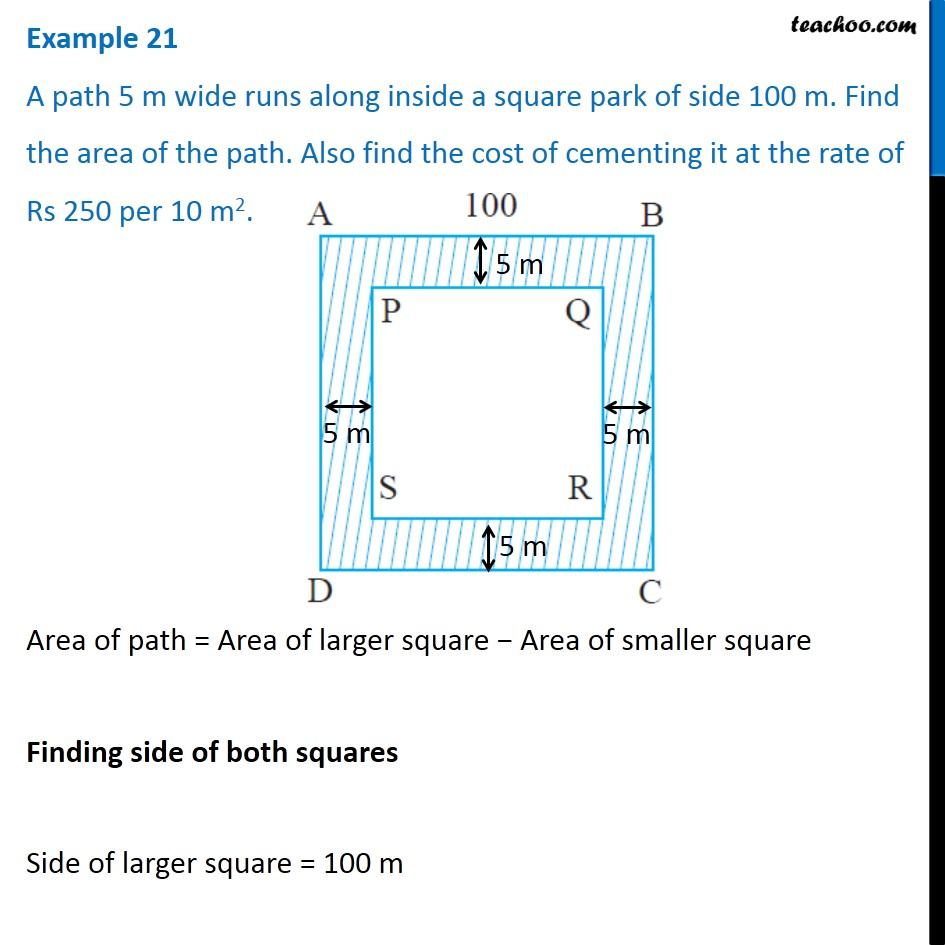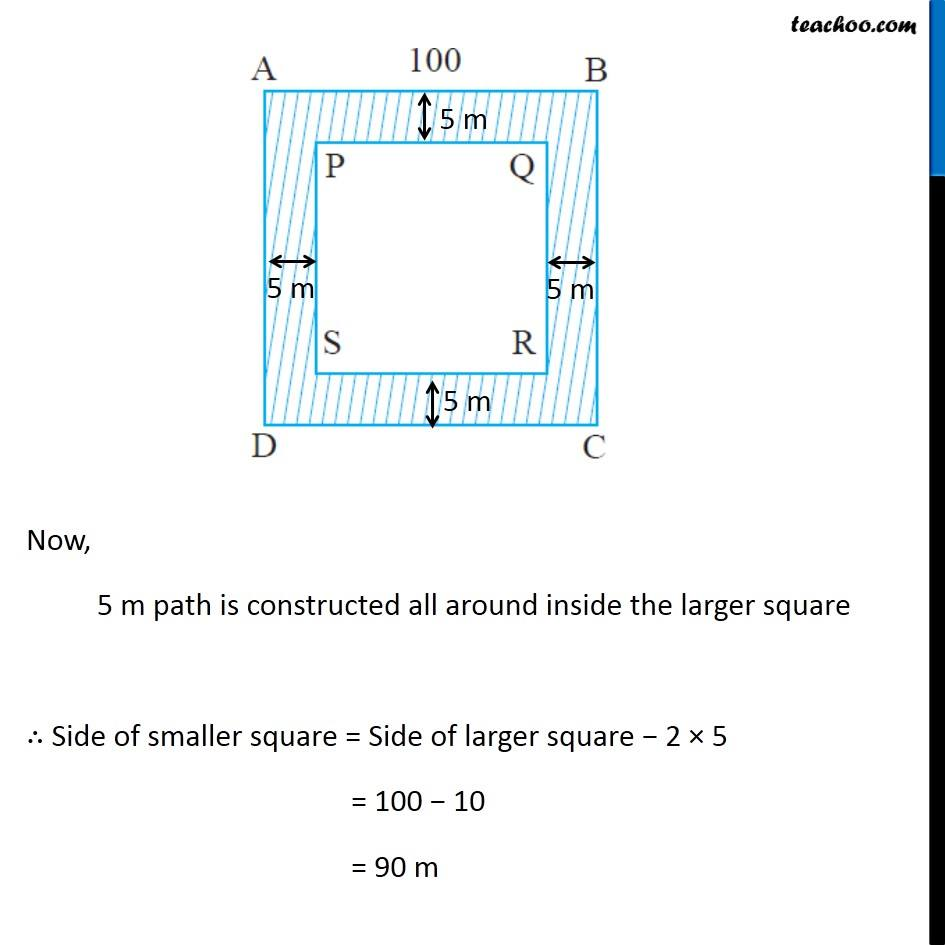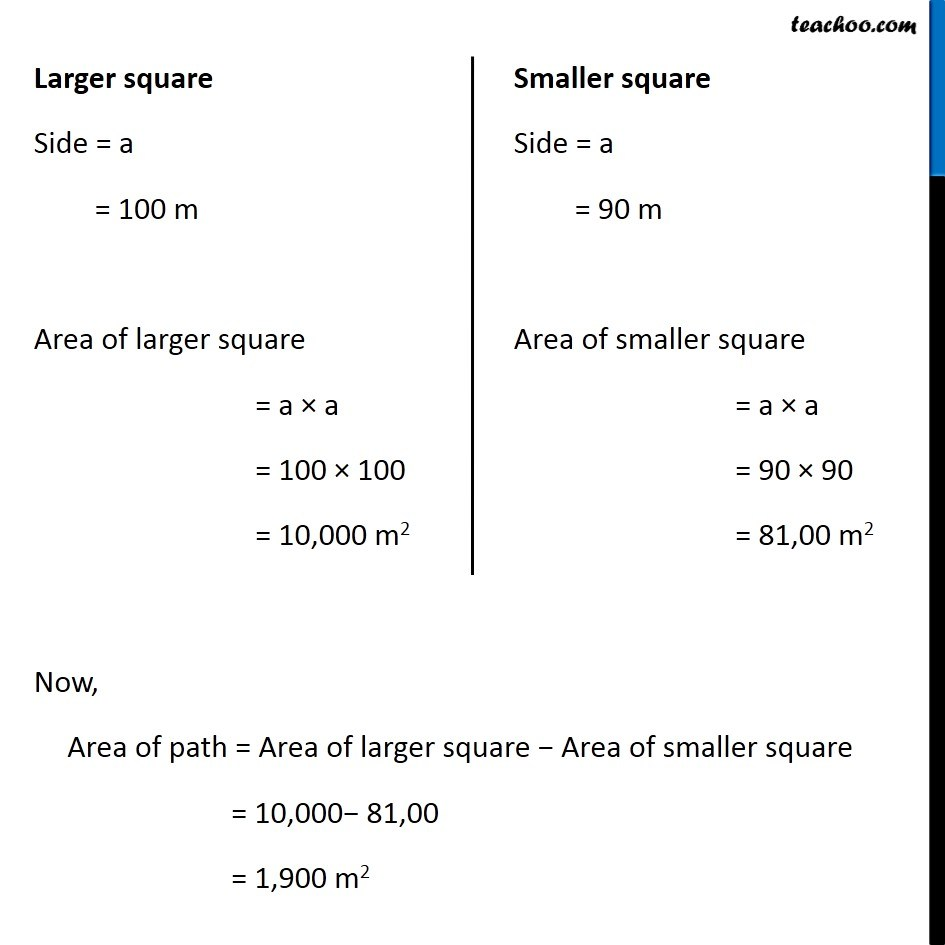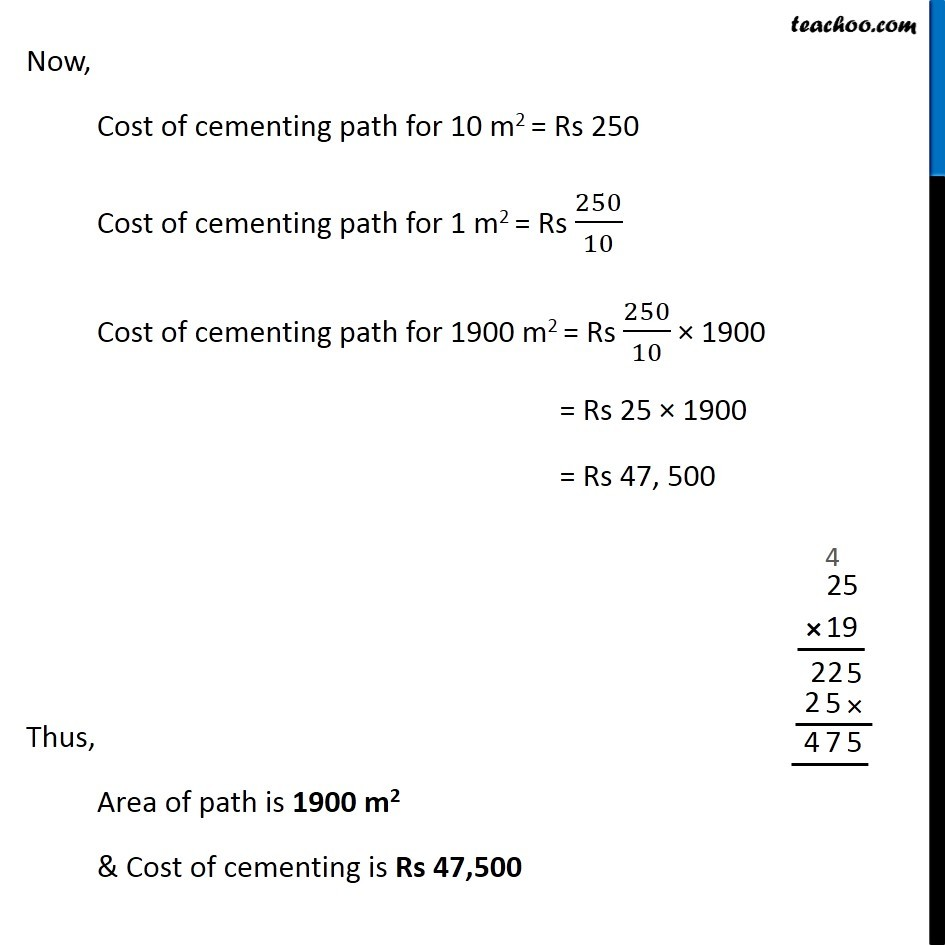1. Chapter 11 Class 7 Perimeter and Area
2. Concept wise
3. Finding Area of rectangle with path outside/inside

Transcript

Example 21 A path 5 m wide runs along inside a square park of side 100 m. Find the area of the path. Also find the cost of cementing it at the rate of Rs 250 per 10 m2. Area of path = Area of larger square − Area of smaller square Finding side of both squares Side of larger square = 100 m Now, 5 m path is constructed all around inside the larger square ∴ Side of smaller square = Side of larger square − 2 × 5 = 100 − 10 = 90 m Larger square Side = a = 100 m Area of larger square = a × a = 100 × 100 = 10,000 m2 Smaller square Side = a = 90 m Area of smaller square = a × a = 90 × 90 = 81,00 m2 Now, Area of path = Area of larger square − Area of smaller square = 10,000− 81,00 = 1,900 m2 Now, Cost of cementing path for 10 m2 = Rs 250 Cost of cementing path for 1 m2 = Rs 250/10 Cost of cementing path for 1900 m2 = Rs 250/10 × 1900 = Rs 25 × 1900 = Rs 47, 500 Thus, Area of path is 1900 m2 & Cost of cementing is Rs 47,500

Finding Area of rectangle with path outside/inside

About the AuthorDavneet Singh
Davneet Singh is a graduate from Indian Institute of Technology, Kanpur. He has been teaching from the past 10 years. He provides courses for Maths and Science at Teachoo.